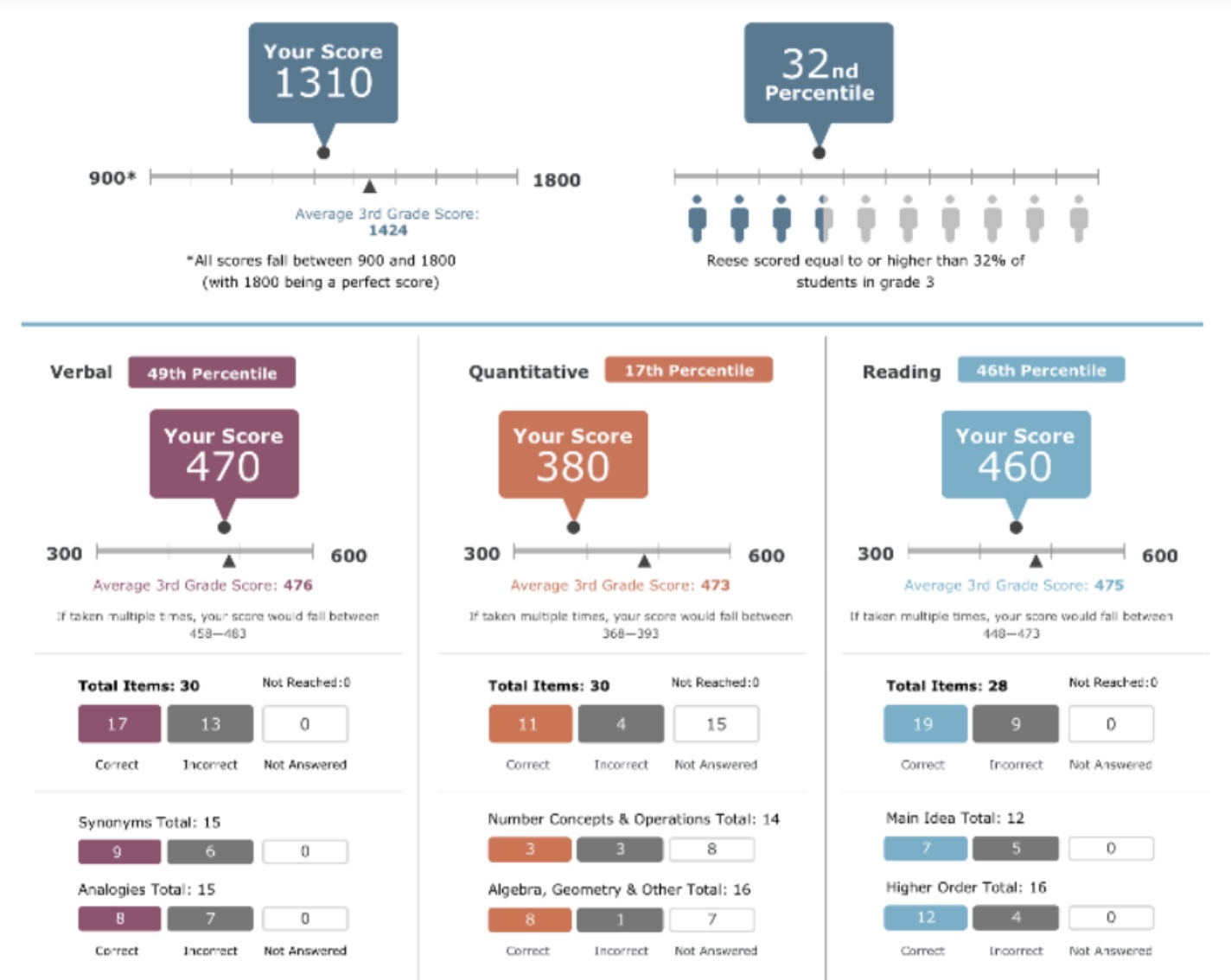Start Now with 100 Free Questions

# How is the SSAT Scored?

At the Elementary Level, the student receives one point for each correct answer and zero points for each incorrect answer. At the Middle and Upper Levels, the student receives one point for each correct answer and subtracts one-quarter of one point for each incorrect answer.

## How the Score is Reported?

### Total Score Summary:

“Your Score” is the total scaled score. This score adds all of the section scores together. The score will be shown on a scale to indicate how the student scored in relation to the lowest and highest possible score. The score for the average student at this grade level will also be posted for comparison. A percentile score will also be shown. This does not show the percent of questions answered correctly but depicts how the student scored in relation to other students taking the same test over the previous three years in the US and Canada. Example: 32nd percentile means that this student scored equal to or better than 32 percent of the students that took the same test.

### Section Scores:

Scores for the verbal, quantitative, and reading sections will also be broken down similar to how the summary score was reported. The scaled score and the percentile score are provided along with the score average for students at the same grade level. A breakdown of the type of question asked and how the student performed in that area is also provided below the section score.

######https://f.hubspotusercontent10.net/hubfs/5232910/SSAT%20Example%20Score%20Reports/SSAT-Elementary-Score-Report-7.21.pdf

Looking for more help to prepare your child for the SSAT? TestingMom has hundreds of practice questions! Try downloading our 100 Free Practice Questions today!